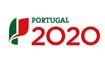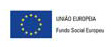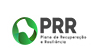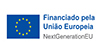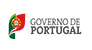# DocenteAleksandar MikovicNome Completo:
Aleksandar Mikovic
ami***@ulusofona.pt
7415-11D5-12E1
0000-0002-0009-1411

• Doutoramento
Física
Matemática

## Publicações

### Artigo em conferência

• Piece-wise flat metrics and quantum gravity , 10-th Mathematical Physics Meeting
• 2006, Spin foam models from the tetrad integration
• 2006, Graviton propagator from spin networks

### Artigo em revista

• 2022-04, Effective Actions for Regge Piecewise Flat Quantum Gravity, Universe
• 2021-03-01, Standard Model and 4-groups, Europhysics Letters
• 2019-01-10, Hamiltonian analysis of the BFCG formulation of general relativity, Classical and Quantum Gravity
• 2018-10-01, Quantum gravity for piecewise flat spacetimes, SFIN
• 2017-02-01, Effective actions for Regge state-sum models of quantum gravity, Advances in Theoretical and Mathematical Physics
• 2016, Hamiltonian analysis of the BFCG theory for the Poincaré 2-group, Classical and Quantum Gravity
• 2015, Solution to the cosmological-constant problem in a Regge quantum gravity model, EPL
• 2015, Canonical formulation of Poincaré BFCG theory and its quantization, General Relativity and Gravitation
• 2014, Categorical generalization of spinfoam models, Journal of Physics: Conference Series
• 2013, Spin-Cube models of quantum gravity, Reviews in Mathematical Physics
• 2013, Preface, Reviews in Mathematical Physics
• 2013, A finiteness bound for the EPRL/FK spin foam model, Classical and Quantum Gravity
• 2012, Poincaré 2-group and quantum gravity, Classical and Quantum Gravity
• 2012, Effective action for EPRL/FK spin foam models, Journal of Physics: Conference Series
• 2011, Lie crossed modules and gauge-invariant actions for 2-BF theories, Advances in Theoretical and Mathematical Physics
• 2011, Large-spin asymptotics of Euclidean LQG flat-space wavefunctions, Advances in Theoretical and Mathematical Physics
• 2011, Four-dimensional spin foam perturbation theory, Symmetry, Integrability and Geometry: Methods and Applications (SIGMA)
• 2011, Effective action and semi-classical limit of spin-foam models, Classical and Quantum Gravity
• 2009, Spin foam perturbation theory for three-dimensional quantum gravity, Communications in Mathematical Physics
• 2008, Spin network wavefunction and nonperturbative graviton propagator, Fortschritte der Physik
• 2008, Invariants of spin networks embedded in three-manifolds, Communications in Mathematical Physics
• 2006, Quantum gravity as a deformed topological quantum field theory, Journal of Physics: Conference Series
• 2006, Quantum gravity as a broken symmetry phase of a BF theory, Symmetry, Integrability and Geometry: Methods and Applications (SIGMA)
• 2006, Erratum: Flat spacetime vacuum in loop quantum gravity (Classical and Quantum Gravity (2004) 21 (3909)), Classical and Quantum Gravity
• 2006, Coherent states expectation values as semiclassical trajectories, Journal of Mathematical Physics
• 2005, New spin foam models of quantum gravity, Modern Physics Letters A
• 2005, Modern Physics Letters A: Preface, Modern Physics Letters A
• 2004, Flat spacetime vacuum in loop quantum gravity, Classical and Quantum Gravity
• 2003, Spin foam models of Yang-Mills theory coupled to gravity, Classical and Quantum Gravity
• 2003, Quantum gravity vacuum and invariants of embedded spin networks, Classical and Quantum Gravity
• 2003, Quantum Field Theory of Open Spin Networks and New Spin Foam Models, International Journal of Modern Physics A
• 2002, Spin foam models of matter coupled to gravity, Classical and Quantum Gravity
• 2001, Super Chern-Simons theory and flat super connections on a torus, Advances in Theoretical and Mathematical Physics
• 2001, Quantum field theory of spin networks, Classical and Quantum Gravity
• 2000, Painlevé III equation and Bianchi VII0 model, Advances in Theoretical and Mathematical Physics
• 2000, Free-field realization of D-dimensional cylindrical gravitational waves, Classical and Quantum Gravity
• 2000, Belinskii - Zakharov formulation for Bianchi models and Painlevé III equation, Journal of Mathematical Physics
• 1999, One-loop corrections for a Schwarzschild black hole via 2D dilaton gravity, Physical Review D - Particles, Fields, Gravitation and Cosmology
• 1998, Remarks on the reduced phase space of (2 + 1)-dimensional gravity on a torus in the Ashtekar formulation, Classical and Quantum Gravity
• 1998, One-loop effective action for spherical scalar field collapse, Classical and Quantum Gravity
• 1998, Free field realization of cylindrically symmetric Einstein gravity, Physics Letters, Section B: Nuclear, Elementary Particle and High-Energy Physics
• 1997, W-strings on curved backgrounds, Modern Physics Letters A
• 1997, One-loop effective action for a generic 2d dilaton gravity theory, Nuclear Physics B
• 1997, On the Dirac-Born-Infeld action for D-branes, Physics Letters, Section B: Nuclear, Elementary Particle and High-Energy Physics
• 1997, Loop corrections in the spectrum of two-dimensional Hawking radiation, Classical and Quantum Gravity
• 1997, General solution for self-gravitating spherical null dust, Physical Review D - Particles, Fields, Gravitation and Cosmology
• 1997, A quantum model of Schwarzschild black hole evaporation, Physics Letters, Section B: Nuclear, Elementary Particle and High-Energy Physics
• 1996, Unitary theory of evaporating two-dimensional black holes, Classical and Quantum Gravity
• 1996, Two-loop back-reaction in 2D dilaton gravity, Nuclear Physics B
• 1995, Hawking radiation and back-reaction in a unitary theory of 2D quantum gravity, Physics Letters B
• 1993, Symplectic structure of 2D dilaton gravity, Physics Letters B
• 1993, Canonical analysis of the Bianchi models in an Ashtekar formulation, Classical and Quantum Gravity
• 1993, 2D dilaton gravity in a unitary gauge, Physics Letters B
• 1992, Hamiltonian construction of W-gravity actions, Physics Letters B
• 1992, Gauge fixing and independent canonical variables in the Ashtekar formulation of general relativity, Nuclear Physics, Section B
• 1992, Exactly solvable models of 2D dilaton quantum gravity, Physics Letters B
• 1992, Ashtekar formulation of (2 + 1)-dimensional gravity on a torus, Nuclear Physics, Section B
• 1991, Gauging the extended conformal algebras, Physics Letters B
• 1991, Fermionic random walk and spinning strings, Physics Letters B
• 1990, Vanishing of the cosmological constant in the green-schwarz superstring theory, Nuclear Physics, Section B
• 1990, Random superstrings, Physics Letters B
• 1990, Covariantly quantizable superparticles, Physics Letters B
• 1989, Covariant vertex operators for the Siegel superstring, Nuclear Physics, Section B
• 1989, BRST charge for the Brink-Casalbuoni-Schwarz superparticle, Physics Letters B
• 1988, On-shell equivalence of superstrings, Physics Letters B
• 1988, Free equations of motion for all D = 6 supermultiplets, Nuclear Physics, Section B
• 1988, Equations of motion for N = 1 massless super-Poincaré representations in D = 3,4 space-time, Nuclear Physics, Section B

### Livro

• 2005, Spin foam models of quantum gravity, Mikovic, A.

### Relatório

• 1997, On the Dirac-Born-Infeld action for D-branes, http://www.scopus.com/inward/record.url?eid=2-s2.0-0030675818&partnerID=MN8TOARS

## Email